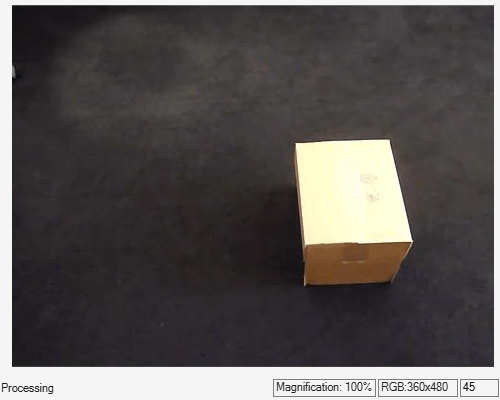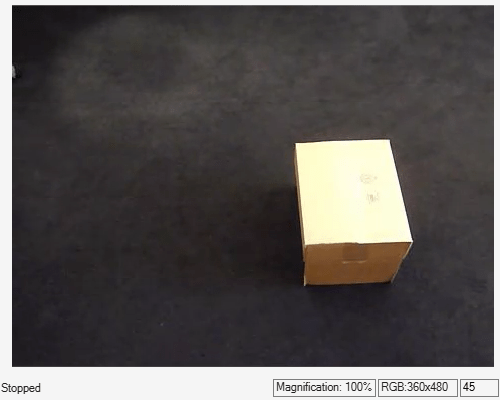# configureKalmanFilter

Create Kalman filter for object tracking

## Syntax

``kalmanFilter = configureKalmanFilter(MotionModel,InitialLocation,InitialEstimateError,MotionNoise,MeasurementNoise)``

## Description

example

````kalmanFilter = configureKalmanFilter(MotionModel,InitialLocation,InitialEstimateError,MotionNoise,MeasurementNoise)` returns a `vision.KalmanFilter` object configured to track a physical object. This object moves with constant velocity or constant acceleration in an M-dimensional Cartesian space. The function determines the number of dimensions, M, from the length of the `InitialLocation` vector.This function provides a simple approach for configuring the `vision.KalmanFilter` object for tracking a physical object in a Cartesian coordinate system. The tracked object may move with either constant velocity or constant acceleration. The statistics are the same along all dimensions. If you need to configure a Kalman filter with different assumptions, use the `vision.KalmanFilter` object directly.```

## Examples

collapse all

Detect and track a ball using Kalman filtering, foreground detection, and blob analysis.

Create System objects to read the video frames, detect foreground physical objects, and display results.

```videoReader = VideoReader('singleball.mp4'); videoPlayer = vision.VideoPlayer('Position',[100,100,500,400]); foregroundDetector = vision.ForegroundDetector('NumTrainingFrames',10,... 'InitialVariance',0.05); blobAnalyzer = vision.BlobAnalysis('AreaOutputPort',false,... 'MinimumBlobArea',70); ```

Process each video frame to detect and track the ball. After reading the current video frame, the example searches for the ball by using background subtraction and blob analysis. When the ball is first detected, the example creates a Kalman filter. The Kalman filter determines the ball?s location, whether it is detected or not. If the ball is detected, the Kalman filter first predicts its state at the current video frame. The filter then uses the newly detected location to correct the state, producing a filtered location. If the ball is missing, the Kalman filter solely relies on its previous state to predict the ball's current location.

``` kalmanFilter = []; isTrackInitialized = false; while hasFrame(videoReader) colorImage = readFrame(videoReader); foregroundMask = step(foregroundDetector,im2gray(im2single(colorImage))); detectedLocation = step(blobAnalyzer,foregroundMask); isObjectDetected = size(detectedLocation, 1) > 0; if ~isTrackInitialized if isObjectDetected kalmanFilter = configureKalmanFilter('ConstantAcceleration',... detectedLocation(1,:), [1 1 1]*1e5, [25, 10, 10], 25); isTrackInitialized = true; end label = ''; circle = zeros(0,3); else if isObjectDetected predict(kalmanFilter); trackedLocation = correct(kalmanFilter, detectedLocation(1,:)); label = 'Corrected'; else trackedLocation = predict(kalmanFilter); label = 'Predicted'; end circle = [trackedLocation, 5]; end colorImage = insertObjectAnnotation(colorImage,'circle',... circle,label,'Color','red'); step(videoPlayer,colorImage); pause(0.1); end ```Release resources.

```release(videoPlayer); ```## Input Arguments

collapse all

Motion model, specified as `'ConstantVelocity'` or `'ConstantAcceleration'`. The motion model you select applies to all dimensions. For example, for the 2-D Cartesian coordinate system. This mode applies to both X and Y directions.

Data Types: `char`

Initial location of object, specified as a numeric vector. This argument also determines the number of dimensions for the coordinate system. For example, if you specify the initial location as a two-element vector, [x0, y0], then a 2-D coordinate system is assumed.

Data Types: `double` | `single` | `int8` | `int16` | `int32` | `int64` | `uint8` | `uint16` | `uint32` | `uint64`

Initial estimate uncertainty variance, specified as a two- or three-element vector. The initial estimate error specifies the variance of the initial estimates of location, velocity, and acceleration of the tracked object. The function assumes a zero initial velocity and acceleration for the object, at the location you set with the `InitialLocation` property. You can set the `InitialEstimateError` to an approximated value:

 (assumed values – actual values)2 + the variance of the values

The value of this property affects the Kalman filter for the first few detections. Later, the estimate error is determined by the noise and input data. A larger value for the initial estimate error helps the Kalman filter to adapt to the detection results faster. However, a larger value also prevents the Kalman filter from removing noise from the first few detections.

Specify the initial estimate error as a two-element vector for constant velocity or a three-element vector for constant acceleration:

MotionModelInitialEstimateError
`ConstantVelocity`[LocationVariance, VelocityVariance]
`ConstantAcceleration`[LocationVariance, VelocityVariance, AccelerationVariance]

Data Types: `double` | `single`

Deviation of selected and actual model, specified as a two- or three-element vector. The motion noise specifies the tolerance of the Kalman filter for the deviation from the chosen model. This tolerance compensates for the difference between the object's actual motion and that of the model you choose. Increasing this value may cause the Kalman filter to change its state to fit the detections. Such an increase may prevent the Kalman filter from removing enough noise from the detections. The values of this property stay constant and therefore may affect the long-term performance of the Kalman filter.

MotionModelInitialEstimateError
`ConstantVelocity`[LocationVariance, VelocityVariance]
`ConstantAcceleration`[LocationVariance, VelocityVariance, AccelerationVariance]

Data Types: `double` | `single`

Variance inaccuracy of detected location, specified as a scalar. It is directly related to the technique used to detect the physical objects. Increasing the `MeasurementNoise` value enables the Kalman filter to remove more noise from the detections. However, it may also cause the Kalman filter to adhere too closely to the motion model you chose, putting less emphasis on the detections. The values of this property stay constant, and therefore may affect the long-term performance of the Kalman filter.

Data Types: `double` | `single`

## Output Arguments

collapse all

Configured Kalman filter, returned as a `vision.KalmanFilter` object for tracking.

## Algorithms

This function provides a simple approach for configuring the vision.KalmanFilter object for tracking. The Kalman filter implements a discrete time, linear State-Space System. The `configureKalmanFilter` function sets the `vision.KalmanFilter` object properties.

 The `InitialLocation` property corresponds to the measurement vector used in the Kalman filter state-space model. This table relates the measurement vector, M, to the state-space model for the Kalman filter. State transition model, A, and Measurement model, H The state transition model, A, and the measurement model, H of the state-space model, are set to block diagonal matrices made from M identical submatrices As and Hs, respectively: A = `blkdiag`(As _1, As _2, ..., As _M)H = `blkdiag`(Hs _1, Hs _2, ..., Hs _M) The submatrices As and Hs are described below: MotionModel As Hs `'ConstantVelocity'` [1 1; 0 1] [1 0] `'ConstantAcceleration'` [1 1 0.5; 0 1 1; 0 0 1] [1 0 0] The Initial State, x: MotionModel Initial state, x `'ConstantVelocity'` [`InitialLocation`(1), 0, ..., `InitialLocation`(M), 0] `'ConstantAcceleration'` [`InitialLocation`(1), 0, 0, ..., `InitialLocation`(M), 0, 0] The initial state estimation error covariance matrix, P: P = `diag`(`repmat`(`InitialError`, [1, M])) The process noise covariance, Q: Q = `diag`(`repmat`(`MotionNoise`, [1, M])) The measurement noise covariance, R: R = `diag`(`repmat`(`MeasurementNoise`, [1, M])).

## Version History

Introduced in R2012b